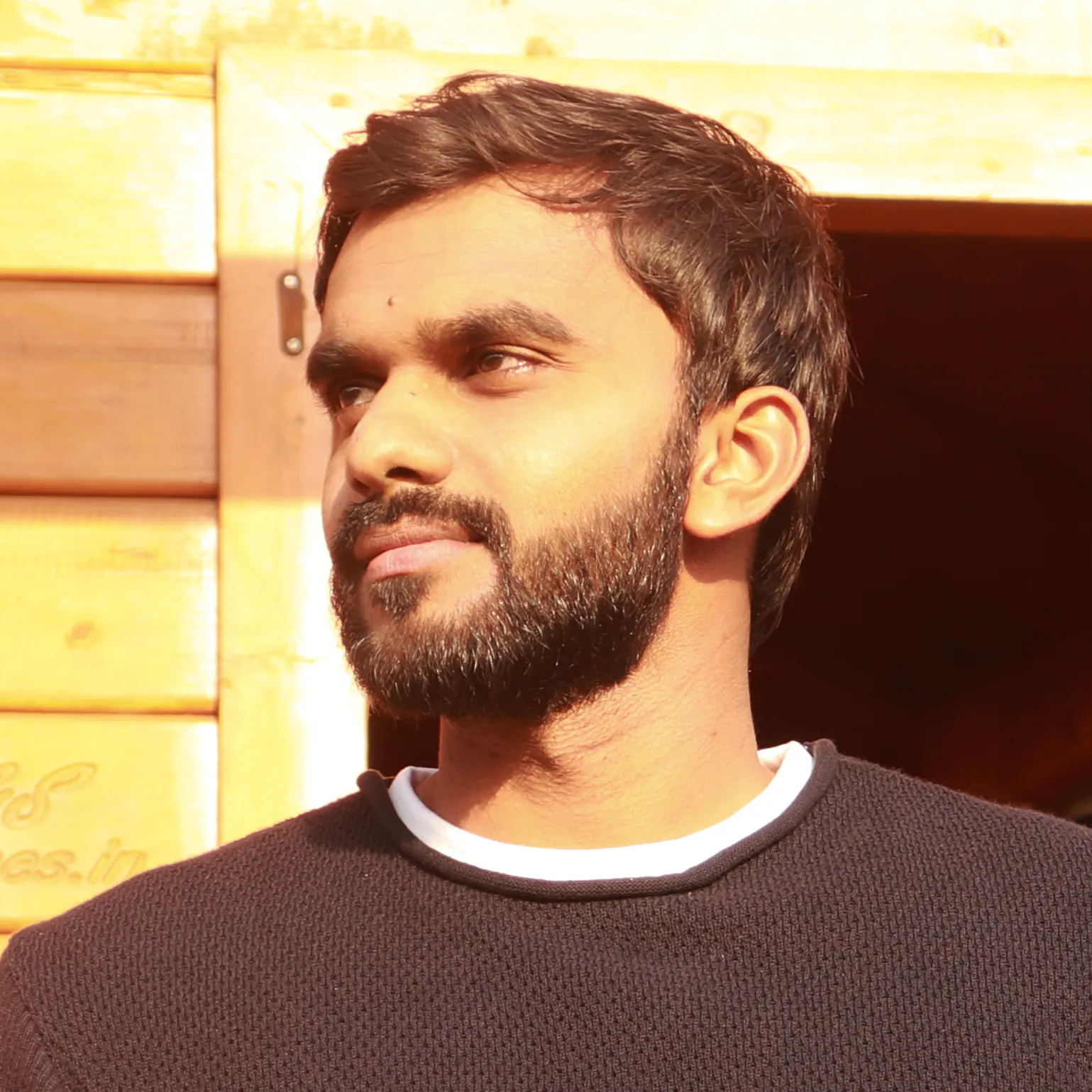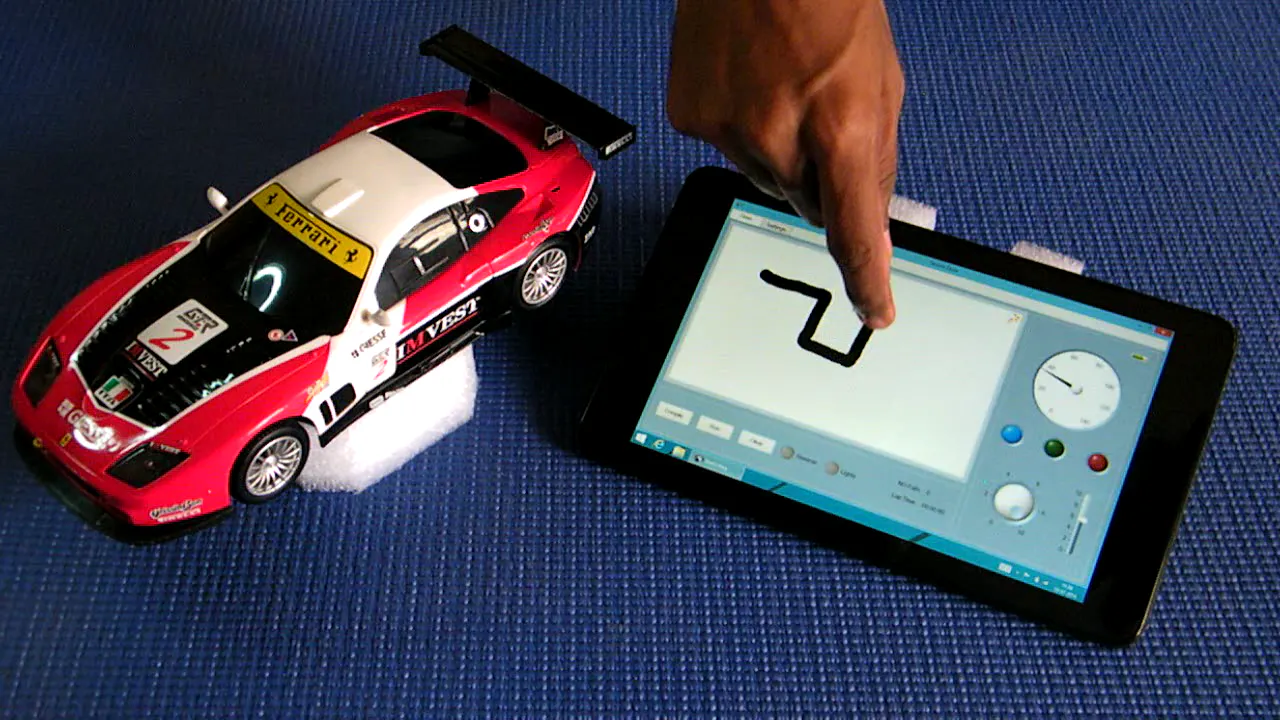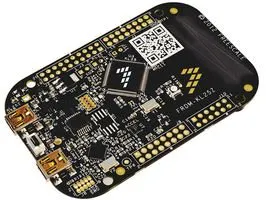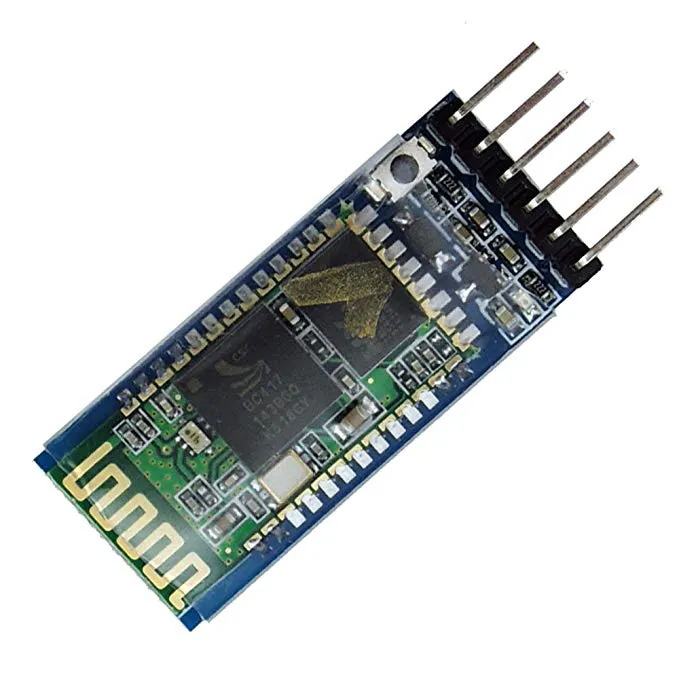# Sketch Drive Car

This project aims at controlling a car through a trajectory traced by the user on a screen running windows or android## Things used in this project

### Hardware componentsNXP FRDM Board
×1HC-05 Bluetooth Module
×1
 L293DNE
×1

### Hand tools and fabrication machines

 CodeWarrior IDE

## Schematics

### Sketch Drive Car Schematic

not updated , iwill update it soon

## Code

### Sketch Drive Car Source

C/C++
```/*
* AppMain.c
*
*  Created on: Mar 9, 2014
*      Author: sony
*/

#include "AppMain.h"
#include "I2C1.h"
#include "AS1.h"
#include "MMA8451.h"
#include "Routing.h"

#define DEBUG

byte bv;
byte DELAY[]= "<DLY00000>";
byte RUN[] = "<RUN00000>";
byte DATA[] = "DAT";
byte TDATA = "<NOP00000>";

void App_Run(void)
{

if(Bat_Voltage < 1.8)
RLED_ON();
else
RLED_OFF();

// Charging Mode Check

#ifndef DEBUG

PackData(DATA,Bat_Voltage,CarAcc.Speed);

GLED_ON();
}
GLED_OFF();
//BLED_ON();
#endif

while(1){

Calibrate();

ParseData();

CarAcc.Speed = GetSpeed();
//preX = AccelX;

PackData(DATA,Bat_Voltage,CarAcc.Speed);

//WAIT1_Waitms(1000);

if(Car.start && Car.mode){

TestDrive();

}

if(Car.start && !Car.mode){

if(istart){
prevDirection = Car.Direction;
istart = false;
}
SetSpeed();

if (prevDirection != Car.Direction){
Car.isTurning = true;
SendString(DELAY);
if(Car.Reverse)
RunReverse();
else
RunDrive();
SendString(RUN);
Car.isTurning = false;
}

}
else{
istart= true;
SetOff();
}

if(Car.lightON){

LLIGHT_ON();
RLIGHT_ON();

}
else
{
LLIGHT_OFF();
RLIGHT_OFF();
}

}

}

void App_Init()
{

BLED_OFF();
LLIGHT_ON();
RLIGHT_ON();

Car.Reverse = 0;
istart = true;
Car.isTurning = false;
Car.isMoving = false;
Car.Speed = 0;
Car.moveBkd = 0;
Car.moveFwd = 0;
Car.moveLeft = 0;
Car.moveRight = 0;
Car.mode = 0;
WAIT1_Waitms(1000);

TurnDly = 1100;
TurnDly = 1200;
TurnDly = 1300;
TurnDly = 1400;
TurnDly = 1500;
TurnDly = 1600;
TurnDly = 1700;
TurnDly = 1800;
TurnDly = 1900;
TurnDly = 2000;

EngSpeed = 40;
EngSpeed = 45;
EngSpeed = 50;
EngSpeed = 55;
EngSpeed = 60;
EngSpeed = 65;
EngSpeed = 70;
EngSpeed = 75;
EngSpeed = 80;
EngSpeed = 85;

CarSpeed = 0;
CarSpeed = 40;
CarSpeed = 44;
CarSpeed = 48;
CarSpeed = 52;
CarSpeed = 56;
CarSpeed = 60;
CarSpeed = 64;
CarSpeed = 68;
CarSpeed = 72;
CarSpeed = 76;
CarSpeed = 80;
CarSpeed = 84;
CarSpeed = 88;
CarSpeed = 92;

LLIGHT_OFF();
RLIGHT_OFF();

}

void SendChar(byte data){

AS1_SendChar(data);

}

void SendString(byte *str) {
while(*str!='\0') {
AS1_SendChar(*str);
str++;
}
}

void SetOff()
{

PWM1_SetDutyMS(0);
PWM2_SetDutyMS(0);

}

{

float voltage;

AD_finished = FALSE; /* reset flag */
/* do something else here... */
}
/* AD_finished set to TRUE by the interrupt to indicate the result is ready */

voltage = (float) ((3.0f * adc_val) / 0xffff);

return voltage;
}

void SetSpeed()
{

if(!Car.Reverse){

PWM1_SetDutyMS(0);
PWM2_SetDutyMS(EngSpeed[Car.EngineSpeed]);
}
else
{

PWM2_SetDutyMS(0);
PWM1_SetDutyMS(EngSpeed[Car.EngineSpeed]);

}

}

void TestDrive(){

if(!Car.Reverse){

PWM1_SetDutyMS(0);
PWM2_SetDutyMS(CarSpeed[Car.Speed/10]);
}
else{
PWM2_SetDutyMS(0);
PWM1_SetDutyMS(CarSpeed[Car.Speed/10]);
}

if(Car.moveLeft && !Car.moveRight ){

TurnLeft();
}

if(!Car.moveLeft && Car.moveRight ){

TurnRight();
}
if(!Car.moveLeft && !Car.moveRight ){

TurnOff();
}

}

void PackData(byte *cmd,float voltage,uint8_t speed)
{
byte volt;

TDATA = '<';
TDATA = cmd;
TDATA = cmd;
TDATA = cmd;

volt = voltage*10;

TDATA = (byte) (volt/10) + '0';
TDATA = (byte) (volt%10) + '0';
TDATA = (byte) (speed/100) + '0';
TDATA = (byte) ((speed/10)%10) + '0';
TDATA = (byte) (speed%10) + '0';
TDATA = '>';

}

void ParseData()
{

//Stream Data Format

//>>>  1010	 32   x 	x	 000000    x       x	        x	    	 x		0	   x	  x	   x    x     x      xxx    x   1010   <<<
//	   ID	 Len  Mode  Dir  Data	   ReEN    EngineSpeed  TurnDelay    start  pause  Light  FWD  BKD  Left  Right  Speed  NA  EID

Car.mode = RxBuf-'0';
Car.Direction = RxBuf-'0';
//Car.Xval = (RxBuf-'0')*100 + (RxBuf-'0')*10 +(RxBuf-'0')*1;
//Car.Yval = (RxBuf-'0')*100 + (RxBuf-'0')*10 +(RxBuf-'0')*1;
Car.Reverse = (bool) (RxBuf-'0') & 0x01;
Car.EngineSpeed = (RxBuf-'0')*1;
Car.TurnDelay = (RxBuf-'0')*1;
Car.start = (bool)(RxBuf-'0') & 0x01;
//Car.stop = (bool)~(RxBuf-'0') & 0x01;
//Car.pause = (bool)(RxBuf-'0') & 0x01;
Car.lightON = (bool)(RxBuf-'0') & 0x01;
Car.moveFwd = (bool)(RxBuf-'0') & 0x01;
Car.moveBkd = (bool)(RxBuf-'0') & 0x01;
Car.moveLeft = (bool)(RxBuf-'0') & 0x01;
Car.moveRight = (bool)(RxBuf-'0') & 0x01;
Car.Speed = (RxBuf-'0')*100 + (RxBuf-'0')*10 +(RxBuf-'0')*1;

}

void Calibrate (void)
{

unsigned int count1 = 0;

do{

CarAcc.sstatex = CarAcc.sstatex + AccelX;  //Accumulate Samples
CarAcc.sstatey = CarAcc.sstatey + AccelY;
count1++;
}while(count1!=0x0400); 	//1024 times

CarAcc.sstatex = CarAcc.sstatex>>10;    //Divide by 1024
CarAcc.sstatey = CarAcc.sstatey>>10;

}

uint8_t GetSpeed(void)
{

unsigned char count2=0;

do{

CarAcc.accelerationx=CarAcc.accelerationx+ AccelX;
CarAcc.accelerationy=CarAcc.accelerationy+ AccelY;
count2++;

}while(count2!=0x40);

CarAcc.accelerationx=CarAcc.accelerationx>>6;  //division by 64
CarAcc.accelerationy=CarAcc.accelerationy>>6;

CarAcc.accelerationx = CarAcc.accelerationx - CarAcc.sstatex; //eliminate zero reference
CarAcc.accelerationy = CarAcc.accelerationy - CarAcc.sstatey; // to obtain positive and negative acceleration

//First X Integeration

CarAcc.velocityx = CarAcc.velocityx + CarAcc.accelerationx + ((CarAcc.accelerationx-CarAcc.accelerationx)>>1);

//First Y Integeration

CarAcc.velocityy = CarAcc.velocityy + CarAcc.accelerationy + ((CarAcc.accelerationy-CarAcc.accelerationy)>>1);

CarAcc.accelerationx = CarAcc.accelerationx;  // The current acceleration value must be sent to previous acceleration

CarAcc.accelerationy = CarAcc.accelerationy;

CarAcc.VX=CarAcc.velocityx-CarAcc.velocityx;
CarAcc.VY=CarAcc.velocityy-CarAcc.velocityy;

CarAcc.velocityx = CarAcc.velocityx;  // The current velocity value must be sent to previous velocity

CarAcc.velocityy = CarAcc.velocityy;

return CarAcc.VX;

}
```

### Sketch Drive Car WinApp.7z

Plain text
`No preview (download only).`

## Credits

### Ashok R

19 projects • 61 followers
Hobbyist/Engineer/Director/Animatior